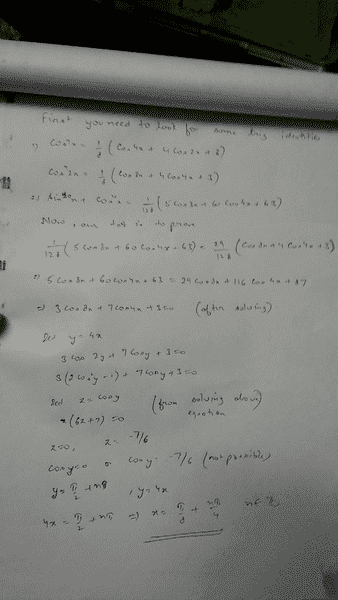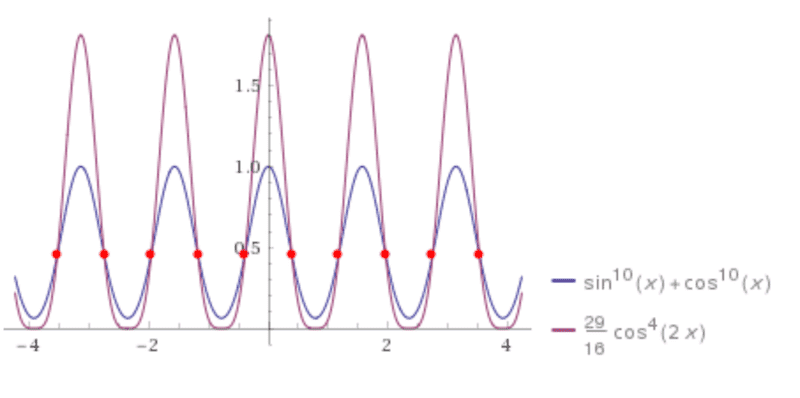# Today, in our class, we received a trigonometric equation

Member has been warned not to delete the template, and to type in his attempt.
Today,in our class, we received a trigonometric equation
##\sin^{10}{x}+\cos^{10}{x}=\frac{29}{16}\cos^4{2x}##

Here is my attempt:#### Attachments

•Delta2

.Scott
Homework Helper
<Moderator's note: Solution removed.>

Here's what it looks like graphed out:#### Attachments

Last edited by a moderator:
•Delta2
.Scott
Homework Helper
I cannot read a lot of your handwriting, but from what I can read, I suspect you do not have it.
I am assuming you are only looking for the real (non complex) roots.

Ray Vickson
Homework Helper
Dearly Missed
Today,in our class, we received a trigonometric equation
##\sin^{10}{x}+\cos^{10}{x}=\frac{29}{16}\cos^4{2x}##

Here is my attempt:
View attachment 227423

Here is one way that will work, but be prepared for pages and pages of algebra---or better yet, use a computer algebra package, as I did: I used Maple.

You can use Euler's formulas (see https://en.wikipedia.org/wiki/Euler's_formula) to write
$$\cos(x) = \frac{1}{2} (u + v), \;\; \sin(x) = \frac{1}{2i} (u - v),$$
where
$$u = e^{ix}, \;\; v = e^{-ix} = \frac 1 u.$$
Also, ##\cos(2x) = (1/2)(e^{2ix} + e^{-2ix}) = (1/2)(u^2 + v^2).## That gives your function
$$f(x) = \sin^{10} (x) + \cos^{10} (x) - \frac{29}{16} \cos^4 (2x)$$
as
$$f = -\left( \frac u 2 - \frac v 2 \right)^{10} + \left( \frac u 2 + \frac v 2 \right)^{10} - \frac{29}{16} \left( \frac{u^2}{2} + \frac{v^2}{2} \right)^4.$$
Expand it all out, set ##v = 1/u##, then simplify. You will get an expression of the form
$$f = -\frac{P(u^4)}{32 u^8},$$
where ##P(y)## is a 4th degree polynomial in ##y## with positive integer coefficients. Furthermore, ##P(y)## factors into two quadratics with integer coefficients, so the equation ##f(x) = 0## translates into the easily solvable equation ##P(y) = 0##, where ##y = u^4 = e^{4ix}.##

ehild
Homework Helper
Today,in our class, we received a trigonometric equation
##\sin^{10}{x}+\cos^{10}{x}=\frac{29}{16}\cos^4{2x}##

Here is my attempt:
View attachment 227423
•